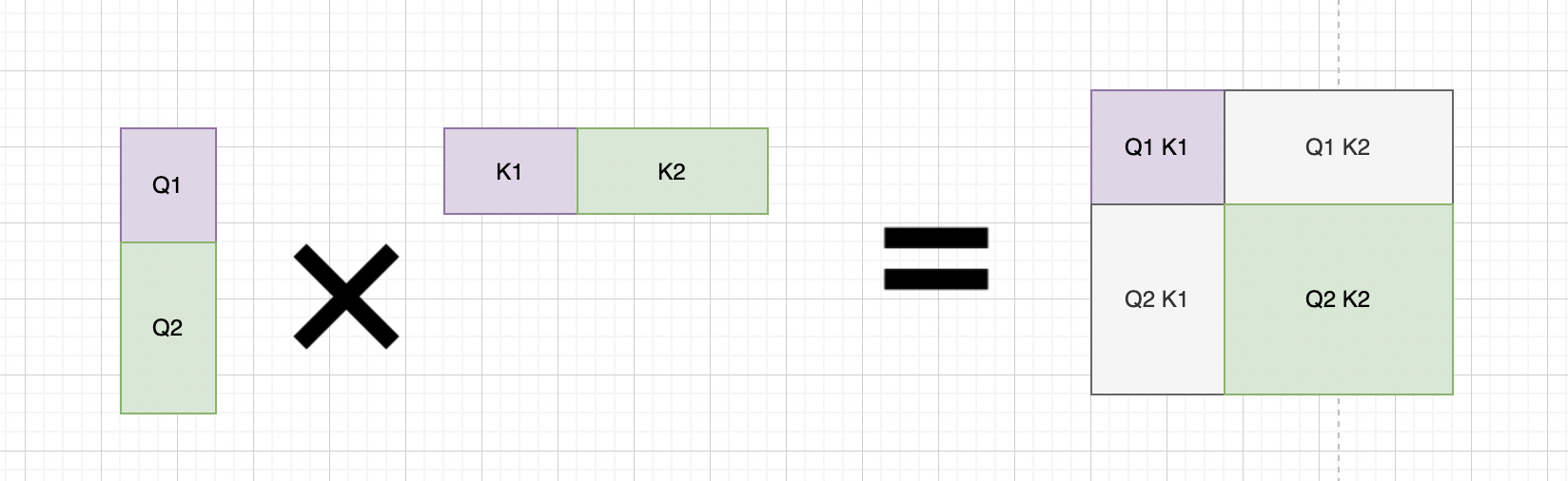# Calculating certain blocks of the output matrix

Hi everyone!

I was wondering if I could only calculate specific entries of a matrix multiplication output. Specifically, I have matrices Q and K (both `N x D`), and I want to calculate a block diagonal sub-matrix of `Q K^T` (The colored part in the figure below):It’s in fact for calculating attention for two (or more) data entries with different number of input features.